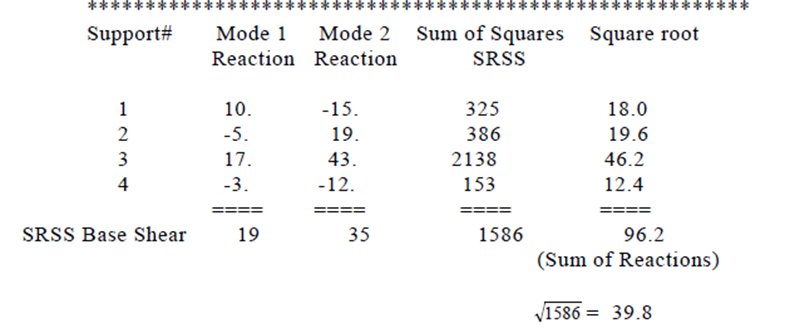# Response Spectrum Analysis in STAAD explanation Video

## Methodology

The design lateral shear force at each floor in each mode is computed by STAAD in accordance with the IS: 1893 (Part 1) -2002

(equation 7.8.4.5c and 7.8.4.5d.)

Qik = Ak*fik*Pk*Wi

## STAAD utilizes the following procedure to generate the lateral seismic loads.

User provides the value for  Z/2xI/R as factors for input spectrum
Program calculates time periods for first six modes or as specified by the user.
Program calculates Sa/g for each mode utilizing time period and damping for each mode.

The program calculates design horizontal acceleration spectrum Ak for different modes.
The program then calculates mode participation factor for different modes.
The peak lateral seismic force at each floor in each mode is calculated.
All response quantities for each mode are calculated.
The peak response quantities are then combined as per method (CQC or SRSS or ABS or TEN or CSM) as defined by the user to get the final results.
The design base shear VB (calculated from the Response Spectrum method) is compared with the base shear Vb (calculated by empirical formula for the fundamental time period).
If VB is less than Vb, all of the response quantities are multiplied by Vb /VB as per Clause 7.8.2.

## How STAAD Calculates of Base Shear in RS Analysis

The base shear, for a given mode for a given direction, reported in the response spectrum analysis is obtained as

VB = X*Y*Z*D

where

X = Mass participation factor for that mode for that direction
Y = Total mass specified for that direction
Z = Spectral acceleration for that mode
D = direction factor specified in that load case

## BMD for RS Load Case

The member forces are computed correctly only at the 2 ends of the member.
The sign of these forces cannot be determined because the method used to combine the contribution of modes cancels the signs.
Force values do not necessarily occur at the same instant of time.
STAAD plots a straight line joining the max. bending moment values at start and end joints of member, absolute (positive) values.

## What is the Scale Factor to be provided when specifying a Response Spectra?

Spectrum data consists of pairs of values which are Period vs. Accn. or Period vs. Displacement.
The acceleration or displacement values obtained for that site may have been provided to you as normalized values or un-normalized values.
Normalization means that the values of acceleration or displacement have been divided by a normalization factor.
One of the commonly used normalization factors is ‘g’, the acceleration due to gravity.

If the spectrum data you specified is normalized, provide the NORMALIZATION FACTOR as the SCALE FACTOR.
If your spectrum data is un-normalized, there is no need to provide a scale factor. Default value of one will be used then.
Make sure that the value you provide for the SCALE FACTOR is matching the length units you have specified. So if the scale factor is ‘g‘ and length is in meter, provide value 9.81 ~ 10.
STAAD will multiply the spectral acceleration or spectral displacement values by the scale factor.

The Direction factor is value by which the spectral displacement for associated direction is multiplied.

SPECTRUM SRSS X 0.7 Y 0.5 Z 0.65 DISP DAMP 0.05 SCALE 32.2

Period is determined for each mode.
Spectral displacement (Sa/g) for that mode is read by interpolating the response spectrum plot.
Spectral displacement “Sa/g” for each direction is multiplied with associated Direction factor.  D
The X direction spectral displacement = “Sa/g” * 0.1822

## How to obtain critical value from the design standpoint?

Create 2 load combination cases for every spectrum case. For example, if the dead load case is 1, and the spectrum load case is 2, you could create

1 1.1 2 1.3

1 1.1 2 -1.3

Use the critical value from amongst these 2 load combination cases for design purposes.
Thus considering a positive effect as well as the negative effect of the spectrum load case.

## Obtain vertical distribution of the total base shear in a RS analysis, like in Static Procedure.

No direct method to obtain lateral force distribution. Since the values from a RS analysis are absolute quantities.
We may add up the shears in the columns above that level for an approximate estimate.

## Global Reaction does not equals Global Base Shear.

• Suppose we have two modes and 4 supports in the x direction & in SRSS combination method the results are computed as follows:Note that SRSS base shear (39.8) does not equal the sum of the SRSS reactions (18.0+19.6+46.2+12.4=96.2).
This is due to the fact that the individual maximums would not occur at the same time and not necessarily with the same sign. So the base shear magnitude is usually much less than the sum of the reactions.

1.2.